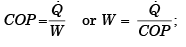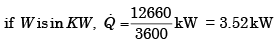Courses

# Test: Heat Pump & Refrigeration Cycles & Systems - 1

## 20 Questions MCQ Test RRB JE for Mechanical Engineering | Test: Heat Pump & Refrigeration Cycles & Systems - 1

Description
This mock test of Test: Heat Pump & Refrigeration Cycles & Systems - 1 for Mechanical Engineering helps you for every Mechanical Engineering entrance exam. This contains 20 Multiple Choice Questions for Mechanical Engineering Test: Heat Pump & Refrigeration Cycles & Systems - 1 (mcq) to study with solutions a complete question bank. The solved questions answers in this Test: Heat Pump & Refrigeration Cycles & Systems - 1 quiz give you a good mix of easy questions and tough questions. Mechanical Engineering students definitely take this Test: Heat Pump & Refrigeration Cycles & Systems - 1 exercise for a better result in the exam. You can find other Test: Heat Pump & Refrigeration Cycles & Systems - 1 extra questions, long questions & short questions for Mechanical Engineering on EduRev as well by searching above.
QUESTION: 1

### A Carnot cycle refrigerator operates between 250K and 300 K. Its coefficient of performance is:

Solution: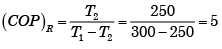QUESTION: 2

### A heat pump works on a reversed Carnot cycle. The temperature in the condenser coils is 27°C and that in the evaporator coils is –23°C. For a work input of 1 kW, how much is the heat pumped?

Solution:

For heat pump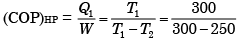or Q1 = 6 × W = 6 kW

QUESTION: 3

### A heat pump for domestic heating operates between a cold system at 0°C and the hot system at 60°C. What is the minimum electric power consumption if the heat rejected is 80000 kJ/hr?

Solution:

For minimum power consumption,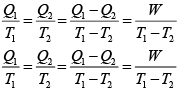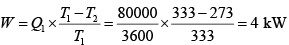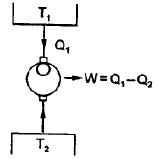QUESTION: 4

In the case of a refrigeration system undergoing an irreversible cycle,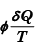is:

Solution:
QUESTION: 5

A heat pump is used to heat a house in the winter and then reversed to cool the house in the summer. The inside temperature of the house is to be maintained at 20°C. The heat transfer through the house walls is 7·9 kJ/s and the outside temperature in winter is 5°C. What is the minimum power (approximate) required driving the heat pump?

Solution: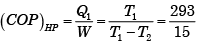orQUESTION: 6

Assertion (A): If a domestic refrigerator works inside an adiabatic room with its door open, the room temperature gradually decreases.

Reason (R): Vapour compression refrigeration cycles have high COP compared to air refrigeration cycles.

Solution:
QUESTION: 7

A building in a cold climate is to be heated by a Carnot heat pump. The minimum outside temperature is –23°C. If the building is to be kept at 27°C and heat requirement is at the rate of 30 kW, what is the minimum power required for heat pump?

Solution: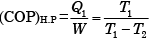or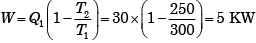QUESTION: 8

A refrigerator working on a reversed Carnot cycle has a C.O.P. of 4. If it works as a heat pump and consumes 1 kW, the heating effect will be:

Solution:

(COP)Heat pump = (COP)refrigerator + 1 = 4 + 1 = 5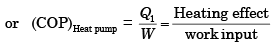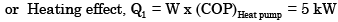QUESTION: 9

Round the clock cooling of an apartment having a load of 300 MJ/day requires an air-conditioning plant of capacity about

Solution:

211 kJ/min = 1 T refrigeration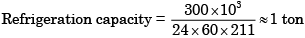QUESTION: 10

A refrigerator based on reversed Carnot cycle works between two such temperatures that the ratio between the low and high temperature is 0.8. If a heat pump is operated between same temperature range, then what would be its COP?

Solution: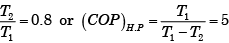QUESTION: 11

A heat pump operating on Carnot cycle pumps heat from a reservoir at 300 K to a reservoir at 600 K. The coefficient of performance is:

Solution: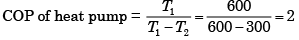QUESTION: 12

The refrigerating efficiency that is the ratio of actual COP to reversible COP of a refrigeration cycle is 0.8, the condenser and evaporator temperatures are 50°C and –30°C respectively. If cooling capacity of the plant is 2.4 kW then what is the work requirement?

Solution:

Condenser Temperature = 273 + 51 = 324 K

Evaporator Temperature = 273 – 30 = 243 K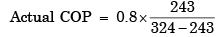∴ We know that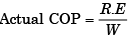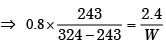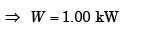QUESTION: 13

A Carnot heat pump works between temperature limits of 277º C and 27º C. Its COP is

Solution: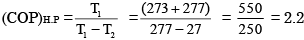QUESTION: 14

Assertion (A ):Power input per TR of a refrigeration system increases with decrease in evaporator temperature.

Reason (R): COP  of  refrigeration system decreases with decrease in evaporator temperature.

Solution:
QUESTION: 15

A Carnot refrigerator requires 1.5 kW/ton of refrigeration to maintain a region at a temperature of – 30°C. The COP of the Carnot refrigerator is:

Solution: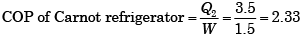QUESTION: 16

The COP of a Carnot refrigeration cycle decreases on

Solution:

COP of Carnot refrigerator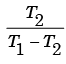will decrease if upper temperature  T1 is
increased and T2 keeping const.

QUESTION: 17

The coefficient of performance of a refrigerator working on a reversed Carnot cycle is 4. The ratio of the highest absolute temperature to the lowest absolute temperature is:

Solution: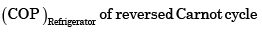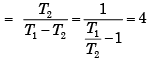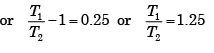QUESTION: 18

Assertion (A): The coefficient of performance of a heat pump is greater than that for the refrigerating machine operating between the same temperature limits

Reason (R): The refrigerating machine requires more energy for working where as a heat pump requires less.

Solution:

R is false. For refrigerating machine our aim is to extract heat from lower temperature source. In heat pump we are interested on heat addition to higher temperature side so it is heat extracted + work added. That so why it’s COP is higher but work requirement is same for both the machine.

QUESTION: 19

The COP of refrigeration cycle is given by

Solution:

Here p1 is the pressure after compression and p2 is the pressure before compression.

QUESTION: 20

The power (kW) required per ton of refrigeration is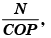where COP is the coefficient of performance, then N is equal to:

Solution: